# Moment of Inertia

• Last Updated : 24 Jun, 2021

The motion of bodies can be of many types like linear motion, circular motion, and rotational motion. According to Newton’s first law of motion, if an object is stationary, it will remain in a stationary state and if it is in motion, it will remain in motion unless an external force is applied to it. This is due to the inertia of the body. Just as linear motion has inertia, so rotational motion has Moment of Inertia. In this article, we are going to study this moment of inertia. Also, we are going to study the definition, mathematical expression, applications and theorem, and more about the moment of inertia. So in a way to learn about the moment of inertia we should know about the basic concept shown below:

Center of Mass

Attention reader! All those who say programming isn't for kids, just haven't met the right mentors yet. Join the  Demo Class for First Step to Coding Course, specifically designed for students of class 8 to 12.

The students will get to learn more about the world of programming in these free classes which will definitely help them in making a wise career choice in the future.

The Center of mass of a body or a system of particles is the point at which the entire mass of that body or system of particles can be assumed to be centered so that the rotational speed of a single particle equal to the total mass of the body-centered at the center of mass is the exact same. As is the motion of the whole body. “The center of the mass of the system is the point which all the mass of the system can be considered to be concentrated“.

Rotational Motion of Rigid Body

If a body rests at a point in such a way that it is free to move around this point, then when an external force is applied to the body, it does not move in the direction of the force but moves along the axis passing through that point starts moving around. this motion of the body is called rotational motion and the axis about which the body rotates is called the rotational axis. “When by applying a force or a couple of forces on a rigid body, that body stars rotating about an axis passing rotating about an axis passing through itself, then its motion is called Rotational Motion“.

### Moment of Inertia

Just as linear motion has inertia, so rotational motion has Moment of Inertia. According to Newton’s first law of motion, an object that is stationary will remain stationary and an object that is in motion will continue to move in a straight line with the same velocity unless an external force is applied to it, that is, an object can’t automatically change its position, but when an external force is applied on the object to change its position, then that object opposes it. This tendency of an object is called its Inertia.

Similarly, everybody in rotational motion, whether it is moving about the axis of rotation or capable of rotating, but at rest, opposes the change caused by the external force. this tendency of the body is called its Moment of Inertia.

Therefore, a body in rotational motion opposes the external force-moment applied to change its rotational state, this tendency is called its Moment of Inertia.

The moment of inertia is a scalar quantity. Mathematically, the product of the square of the mass of a particle and the distance from the axis of rotation is called the moment of inertia of the particle about the axis of rotation.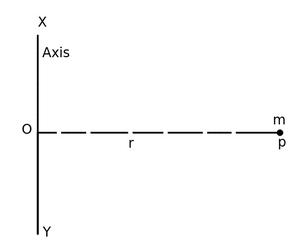Suppose a particle of mass m is moving with respect to the rotational axis XY, then the moment of inertia of the particle about XY will be I = mr2

Expression for Moment of Inertia of a body

Let a rigid body be moving with a uniform angular velocity ω about an axis AB passing through a point O perpendicular to the plane. Suppose this body is made up of many small particles whose mass is m1, m2, m3, ….etc. and their distances from the axis of rotation are respectively r1, r2, r3…., etc.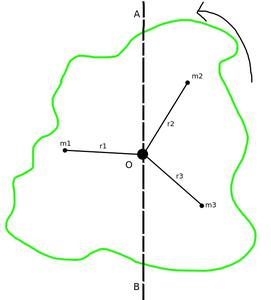By the definition, the moment of inertia of a particle about the axis AB = The mass of the particle * Square the distance of the particle from the axis of rotation

So, Moment of inertia of first particle = m1*r12

Moment of inertia of first particle = m2*r22

Moment of inertia of first particle = m3*r32

………………………………………..Etc.

Now the moment of inertia of the entire body about the axis of rotation AB will be equal to the sum of the moment of inertia of all the particles, so

I = m1*r12 + m2*r22 + m3*r32 +……

or I = Σ m*r2

Here I represent the moment of inertia of the whole body about the axis of rotation and the sign Σ represents the sum. It is clear from the equation that the moment of inertia of a body about an axis of rotation is equal to the sum of the product of the mass of each particle of that body and the square of its perpendicular distance from the axis of rotation.

Note: After the change in the size or shape or axis of the body, the moment of inertia will be changed.

The radius of gyration of a body is the perpendicular to distance from the axis of rotation to the point at which the moment of inertia obtained by taking the total mass of the body as the center is equal to the actual moment of inertia of the object. It is denoted by K.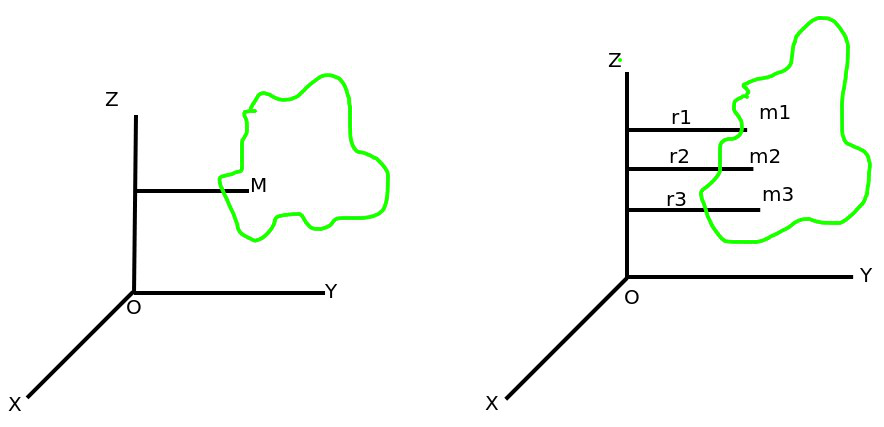If the mass and radius of gyration of the body is M and K respectively, then the moment of inertia of a body is

I = MK2 ……1

Thus, the radius of gyration of a body is that perpendicular to the axis of rotation whose square multiplied by the mass of that body gives the moment of inertia of that body about that axis.

Again by the equation 1, K2 = I/M

or K = √I/m

Thus, the radius of gyration of a body about an axis is equal to the square root of the ratio of the body about that axis.

### Theorems on Moment of Inertia

There are three types of theorems that are very important with respect to Moment of Inertia:

2. The theorem of Vertical axis and
3. The theorem of the Parallel axis.

The moment of inertia of a system composed of many particles about a single axis of rotation is equal to the sum of the moments of inertia of each particle about that axis. if I1, I2, I3……..etc are respectively the moment of inertia of different particles of the system about the same axis of rotation, then the total moment of inertia of the system.

I = I1 + I2 + I3 + ……………….

Clearly, if a piece of the moment of inertia I’ is removed from a body having a moment of inertia I, then the remaining body will have a remaining moment of inertia = (I – I’) about the same axis of rotation.

Theorem of Vertical Axis

The sum of the moment of inertia of a body about two mutually perpendicular axes situated in the plane of a body is equal to the moment of inertia of the body about the third axis which is perpendicular to the two axes and passes through their point of intersection.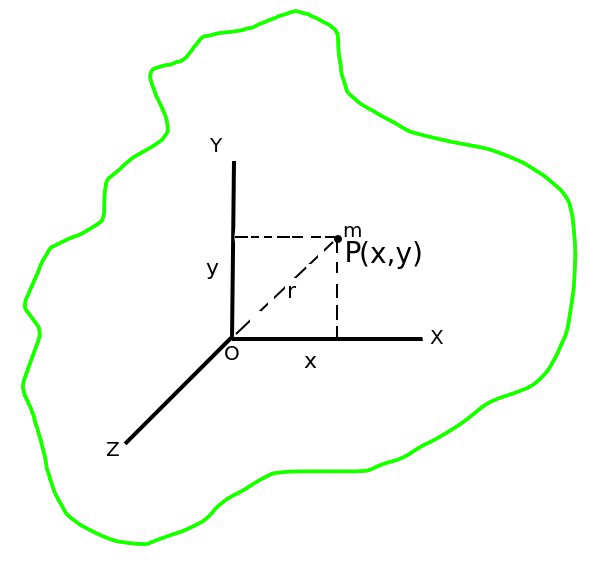In the above figure. OX and OY are two such axes in the plane of the body which are perpendicular to each other. The third axis is OZ which is perpendicular to the plane of the body and passes through the point of intersection of the OX and OY axes. If Ix, Iy, and Iz are the moments of inertia of the body about the axis OX, OY, and OZ axes respectively, then according to this theorem

Ix + Iy = Iz

Theorem of Parallel Axis

According to this theorem, the moment of inertia of a body about a given axis is the sum of the moment of inertia about an axis passing through the center of mass of that body and the product of the square of the mass of the body and the perpendicular distance between the two axes.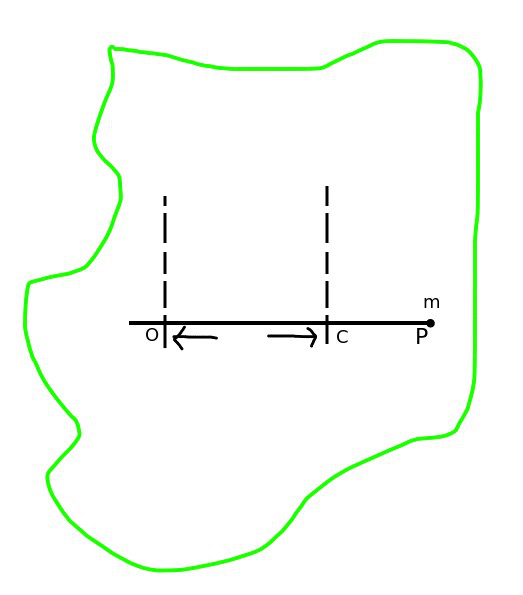Let in the above figure, we have to find the moment of inertia of IO of the body passing through the point O and about the axis perpendicular to the plane, while the moment of inertia of the body passing through the center of mass C and about an axis parallel to the given axis is IC, then according to this theorem

IO = IC + Ml2

where M is the mass of the entire body and l is the perpendicular distance between two axes.

### Moment of Inertia of Some Regular Bodies

• Solid Rectangular Plate: If the mass of the plate is M, length l, and width b, then the moment of inertia passing through the center of gravity and about an axis perpendicular to the plane of the plate.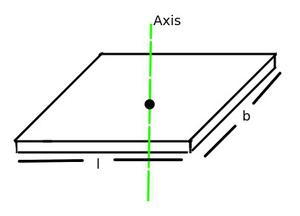I = M( l2 + b2 / 12)

• Circular Disc: If the disc has a mass M and radius r, then the moment of inertia about the disc’s geometric axis is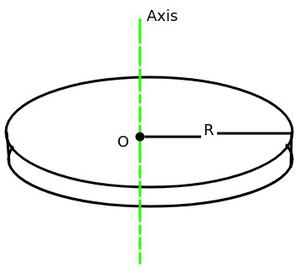I = 1/2(Mr2)

• Uniform Thin Rod: If the mass of the rod is M and the length is l, then the moment of inertia about the axis perpendicular to the length of the rod and passing through its center of gravity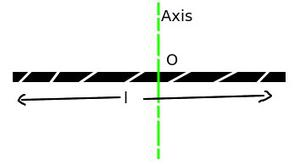I = Ml2/12

• Circular Ring: If the mass of the ring M and radius of the ring is r, then the moment of inertia about the axis passing through perpendicularly to the center of the ring is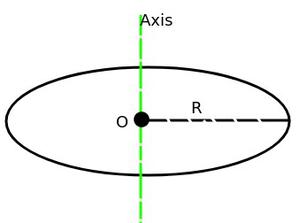I = Mr2

• Solid Sphere: If Solid Sphere has the mass of M and radius r, then the moment of inertia about its diameter is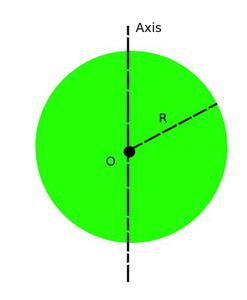I = 2/5Mr2

Difference Between Moment of Inertia and Inertia

Application of Moment of Inertia

1. Due to the greater moment of inertia, the earth is rotating on its axis with the same angular velocity.
2. A small moving wheel is placed under the children’s play motor. After rubbing this wheel with the ground and leaving the motor, due to the moment of inertia of the wheel, the motor keeps running for some time.
3. Each engine consists of a large and heavy wheel attached to its shaft, with most of its mass on its circumference. Therefore, its moment of inertia is high. This wheel is called a flywheel. The torque that drives the shaft of the engine keeps on increasing. Therefore, the rotation of the shaft may not be uniform, but due to the presence of a moving wheel with more inertia, the shaft continues to rotate with almost uniform speed.
4. In the wheel of bullock cart, rickshaw, scooter, cycle, etc., most of the mass is concentrated on its circle or rim. this hoop or routine is attached to the axis of the wheel by rigid spokes. By doing this its moment of inertia is increases. Therefore, when the legs stop moving while cycling, the wheel continues to spin for some time.

### Sample Problems

Question 1. A body of mass 500 g is rotating about an axis. the distance of the center of mass of the body from the axis of rotation is 1.2 m. find the moment of inertia of the body about the axis of rotation.

Solution:

Given that M = 500 g = 0.5 kg, r = 1.2 m.

Obviously, the entire mass of a body can be assumed to be placed at its center of mass. Then the moment of inertia of the body about the axis of rotation.

I = Mr2

=> I = 0.5 * (1.2)2

=> I = 0.72 kg m2

Question 2. The radius of revolution about an axis 12 cm away from the center of mass of a body of mass 1.2 kg is 13 cm. calculate the radius of revolution and moment of inertia about an axis passing through the center of mass.

Solution:

Given that, M = 1.0 kg, K = 13 cm, l = 12 cm, KCM = ?, ICM = ?

From Theorem of Parallel Axis I = ICM + Ml2

=> K2 = KCM2 + l2

or KCM2 = K2 – l2

=> KCM2 = (13)2 – (12)2 = 25

=> KCM = 5

Now, Moment of Inertia ICM = MKCM2

ICM = 1.0 * (0.05)2 = 2.5 * 10-3 kg m2

Question 3. A body of mass 0.1 kg is rotating about an axis. if the distance of the center of mass of the body from the axis of rotation is 0.5 m, then find the moment of inertia of the body.

Solution:

Given that, M = 0.1 kg and r = 0.5 m

so I = Mr2

=> I = 0.1 * (0.5)2

=> I = 0.025 kg m2

Question 4. The moment of inertia of the rings about an axis passing through its center perpendicular to the plane of the circular ring is 200 gm cm2. What will be the moment of inertia about its diameter?

Solution:

Moment of Inertia of a circular ring about an axis passing through another center perpendicular to its plane

MR2 = 200 gm cm2

Moment of inertia about to diameter

= 1/2 MR2

= 1/2 * 200 = 100 gm cm2

Question 5. On what does the moment of inertia of a body depend?

Solution:

The moment of inertia depends on the below factors:

1. Mass of the Body,

2. Distribution of mass of body, and

3. At the position of the rotation-axis.

Keep in mind that changing the shape or size of the body or the axis of rotation changes its moment of inertia.

My Personal Notes arrow_drop_up